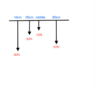# Finding the center of gravity with torque

ari-anne
Homework Statement:
A uniform meter stick of weight 10N is loaded as follows: an 80N weight attached at the 10cm mark, a 30N weight attached at the 20cm mark, a 50N weight attached at the 60cm mark. What is the center of gravity of the loaded meter stick?
Relevant Equations:
Torque= force x distance(when perpendicular)
I don't know how to find the center of gravity much less in this problem, so I just created a diagram of the pole with all the forces.

Homework Helper
Gold Member
2022 Award
Homework Statement:: A uniform meter stick of weight 10N is loaded as follows: an 80N weight attached at the 10cm mark, a 30N weight attached at the 20cm mark, a 50N weight attached at the 60cm mark. What is the center of gravity of the loaded meter stick?
Relevant Equations:: Torque= force x distance(when perpendicular)

I don't know how to find the center of gravity much less in this problem, so I just created a diagram of the pole with all the forces.

Let the centre of mass be at the x cm mark and compute the torques about it.

ari-anne
I placed the weight in where I thought it might go because it doesn't specify in the problem where the end points are or the center of the pole is

#### Attachments

•Screen Shot 2020-02-06 at 11.03.17 PM.png
5.2 KB · Views: 138